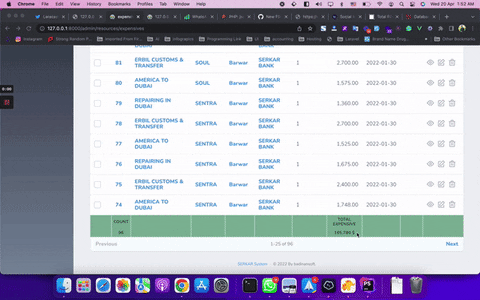17

Stars

2

Version

1.0.1

# Table footer

This Laravel Nova package is used for calculating the total of every column that supports every function you need.## Requirements

• `php: >=7.4`
• `laravel/nova: ^3.0`

## Features

• Add a footer to any index table you want.
• Any calculatable columns you want you can get total
• Support this function
• sum
• count
• avg
• min
• max

## Screenshot## Installation

Install the package in a Laravel Nova project via Composer:

``````composer require badinansoft/table-footer
``````

## Usage

To use these packages just need to install the package by the above command then for any resource any index filed add this method:-

``````     ID::make(__('ID'), 'id')->calculate('count',__('Total Count')),
``````

By above code in the footer of the ID column will show the total count of the id's The ` calculate(\$function,\$label,\$symbol='')` accept 3 argument as you see also support localization 2 arguments are required but \$symbol is not required just for adding the symbol of the currency end of the amount

``````     Number::make(__('Amount'),'amount')
->calculate('avg',__('Average Amount'),'\$'),
``````